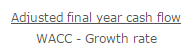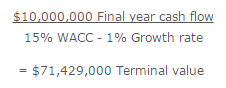Accounting

# What is Present Value of Perpetuity?

The perpetuity concept refers to an infinite series of identical cash flows. It is most commonly applied to a discounted cash flow analysis, where this stream of cash flows is discounted to its present value. The specific application is to the aggregation of all cash flows beyond the date range for which more precise cash flows are being predicted, which is called the terminal value of a project.

Terminal value can be calculated with the perpetuity formula, which employs the following steps:

• Estimate the cash flows associated with the final year of projections, and eliminate from this amount any unusual items that are not expected to occur again in later years.
• Estimate a reasonable growth rate for this adjusted cash flow figure for later years. The amount should approximate the rate of growth for the entire economy. The rate of sustainable growth should be quite small, and may even be zero or a negative figure.
• Subtract this growth rate from the company’s weighted-average cost of capital (WACC), and divide the result into the adjusted cash flows for the final year. The formula is:For example, Glow Atomic is reviewing the projected income stream from a new type of fusion plant that could generate electricity in perpetuity. The analysis is broken into annual cash flows for the first 20 years, followed by a terminal value. The expected cash flow for the 20th year is \$10,000,000. Glow expects these cash flows to increase at a rate of 1% thereafter. The company has a 15% WACC. Based on this information, the terminal value of the investment opportunity is:The present value of a perpetuity can change if the discount rate changes. For example, if the discount rate declines, this will increase the present value, and vice versa.